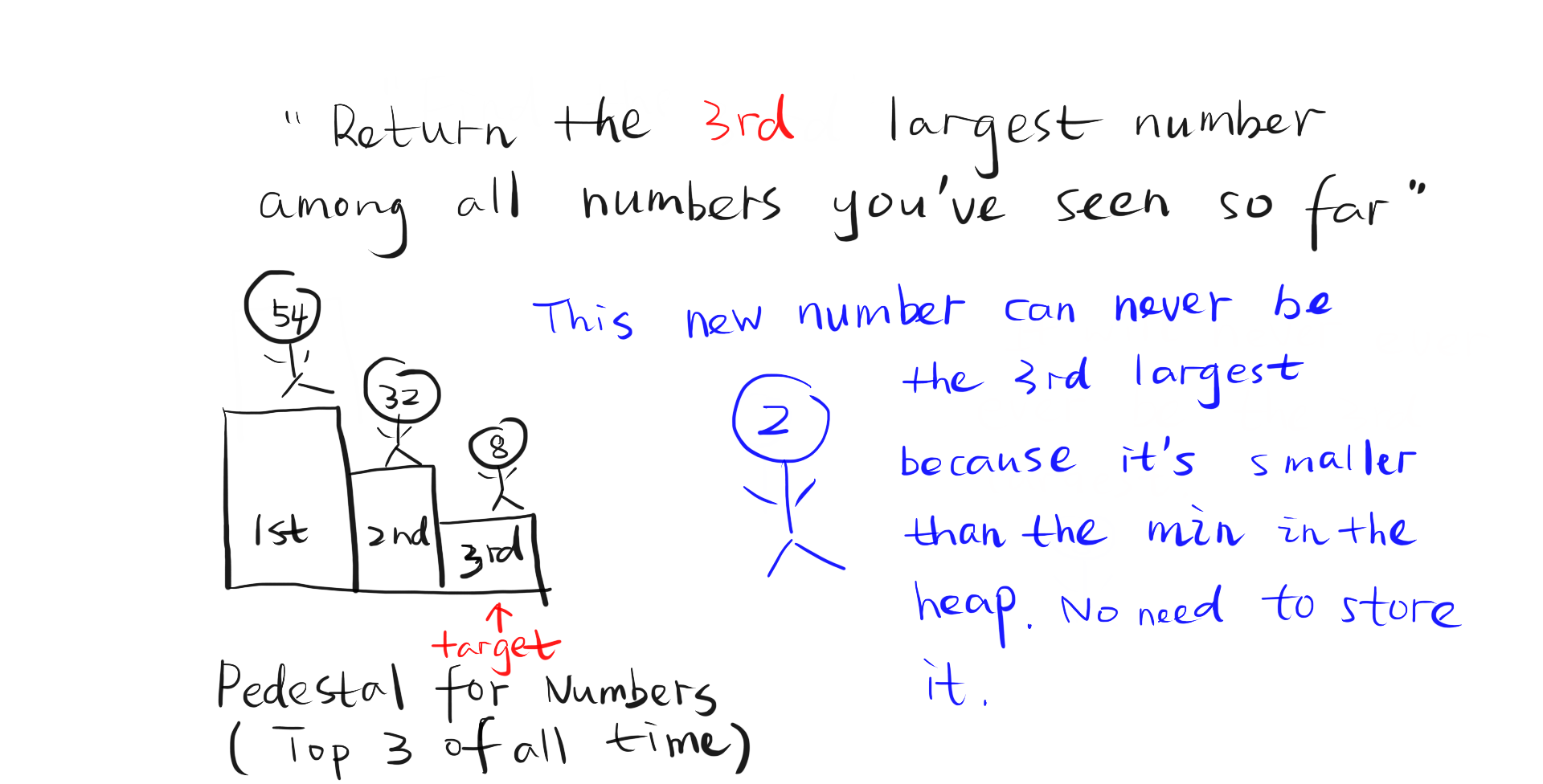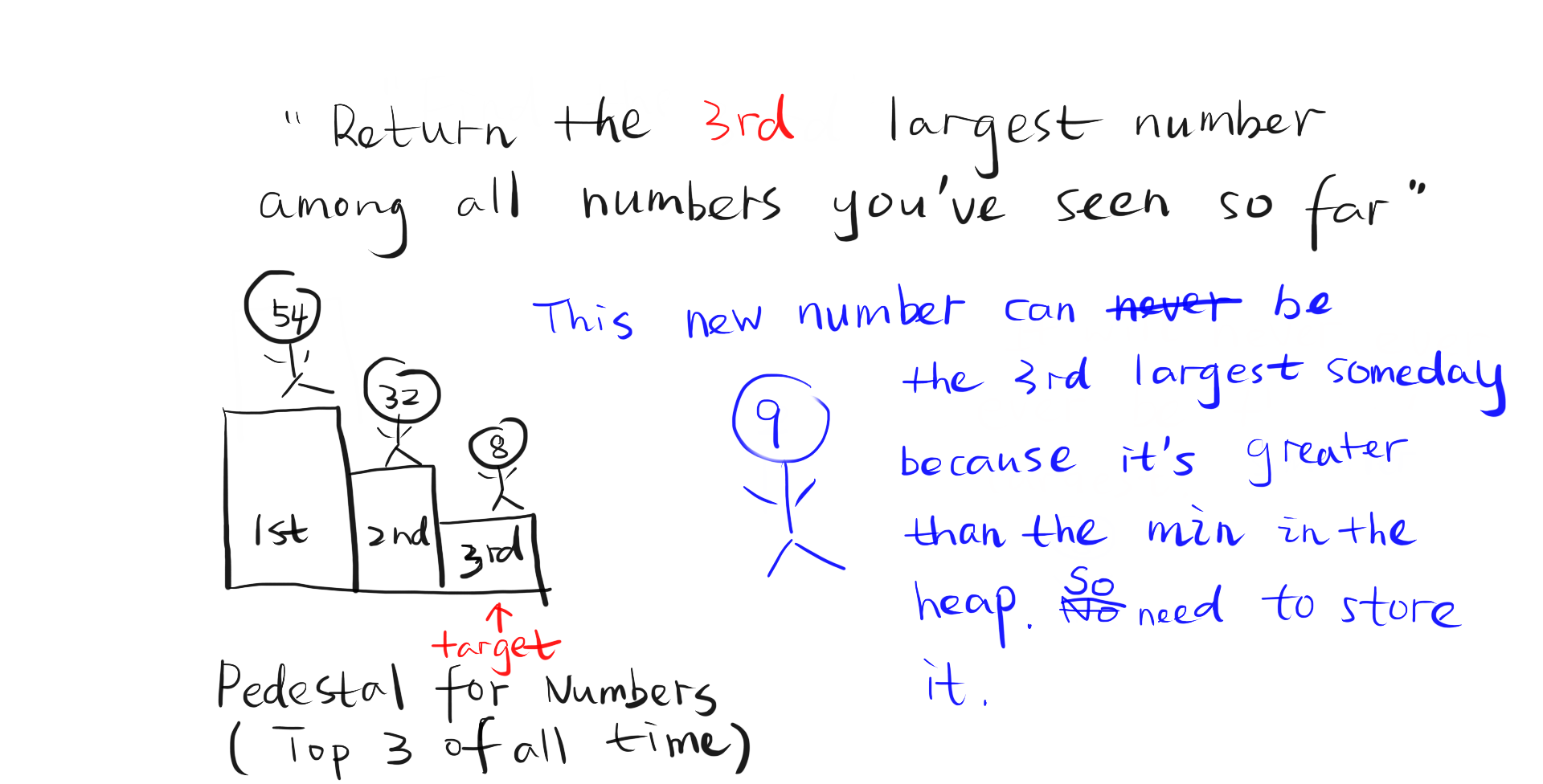# A Very Thorough Solution to Sliding Window Median and Some Heap MagicsThis image is not particularly packed with information. It is just inserted becaue pictures are more fun.

The Sliding Window Median problem has a deceptively simple name, but its solution is definitely not as simple as the name implied. This article aims to provide a very thorough solution to the problem and considerations of all related concepts. Hopefully, this is the last article you need to read about this kind of problems and it is as informative as you want. Let’s get started!

The problem definition is as follows:

Median is the middle value in an ordered integer list. If the size of the list is even, there is no middle value. So the median is the mean of the two middle value.

Examples:
[2,3,4] , the median is 3

[2,3], the median is (2 + 3) / 2 = 2.5

Given an array nums, there is a sliding window of size k which is moving from the very left of the array to the very right. You can only see the k numbers in the window. Each time the sliding window moves right by one position. Your job is to output the median array for each window in the original array.

A very obvious solution will be to sort the k numbers within a sliding window for each iteration. Suppose the length of the array is n, the total time complexity will be O(nklogk).

Of course we can do better than that! We notice that when the sliding window moves one step, a new number is added to the window and an old number is removed from the window. Therefore, for each iteration, we do not need to sort the whole sliding window again. We just need to remove the old number (O(k) time) and insert the new number at the correct position (O(k) time) of the already sorted window. Therefore, the total time complexity will be O(nk). I did not test this particular algorithm on leetcode, but I tested another O(nk) algorithm and it passed the test. Although an O(nk) algorithm is good enough for leetcode, it is not good enough for us truth-seeking perfection-pursuing indoor-dwelling activity-lacking human beings!

Can we still do better? Yes! Now enters the secret ingredient — heap! Heap is particularly good at dynamically tracking something like the x-th largest number. Imagine that a stream of numbers is coming to you and you need to return the x-th largest number of all the numbers you have seen so far when each new number is encountered. This scenario is dynamic because you need to find the answers (x-th largest number) many times over constantly changing data. The solution is using a min heap to store the top x largest numbers and the top of the heap is the x-th largest number. When a new number comes, if it is smaller than the top of the heap, it can be discarded because it will never be the x-th largest number. If the new number is greater than the top of the heap, it will be added to the heap and the old top of the heap will be popped out.when new number is smaller than the top of the min heapwhen new number is greater than the top of the min heap

A median of k numbers is just roughly the k/2-th smallest number so it seems that a heap can be used here. In this scenario, we need two heaps instead of only one heap in the previous scenario. In the previous scenario, in order to find the x-th largest number, only the new numbers greater than the old x-th largest number have to be recorded, so only one heap is needed. In order to find the median of the sliding window, both new numbers greater than the old median and new numbers smaller than the old median need to be recorded, because both kinds of new numbers are candidates for the median.

Since two heaps are needed and the median number(s) has to be at the top of the heap for easy access, the ultimate data structure is easy to guess — a max heap storing the smaller half of numbers in the sliding window and a min heap storing the larger half of numbers, as illustrated in the image below. The top numbers of the heaps are what we need to compute the median. When the length of the sliding window is an even number, size of max heap is the same as size of min heap. When it is an odd number, max heap will have one more item than min heap.

Now that we know the data structure we want, it is time to design an algorithm to maintain this data structure. Maybe the following content would seem too trivial and obvious to you. Please bear with me because I am trying to re-create the problem solving process instead of jumping straight to the solution like it just fell out of the sky. The first thing we know is that we need a loop that moves the sliding window. Ok. Great first step. Now what will happen in each iteration of the loop?

Before we tackle this question, it is helpful to think about the loop invariants. Loop invariants are qualities that do not change during the execution of the whole loop. In this problem, the loop invariants are: 1. All numbers in the sliding window and only those should be in the two-heap stucture. 2. The maximum of the max heap ≤ the minimum of the min heap. 3. size of max heap = size of min heap or size of min heap + 1.

In order to maintain these loop invariants, each iteration should: 1. Add a new number encountered by the sliding window to the two-heap structure and remove an old number the sliding window just left from the structure. 2. If new number ≤ maximum of max heap, add it to the max heap; otherwise, add it to the min heap. 3. Maintain the size relationship between the two heaps by popping items from the heap that has too many items and pushing them in the other heap. Now we got the complete algorithm. The total time complexity is O(nlog(size of heap)) = O(nlogk).

An illustration of balancing the sizes of two heaps (popping the top item from one heap and pushing it into the other) is as follows:

Since the algorithm design is done, now comes time for implementation, which will be coded in Python. The heap in Python is a simple Python list plus heap functionalities supported in the heapq standard library. The problem with this heapq library is that although the theoretical time complexity for removing an item at any position of the heap is O(log(size of heap)), this kind of removal is not supported in the heapq library. The only way to remove an item at an arbitrary position is to loop through the whole heap to remove that item and to call heapq.heapify(heap), which make time complexity deteriorate to O(size of heap).

There are two solutions to achieve the theoretical time complexity of O(log(size of heap)): 1. implementing a hash-heap data structure; 2. using the lazy deletion technique.

A hash-heap data structure is a standard heap plus a hashing table mapping the value of an item to the index of the item.

The lazy deletion technique means that when items need to be removed, it just records the items but does not actually remove them. Actual deletions only happen when the top of the heap is queried and the real top of the heap is blocked by items that should have been removed. Only at that time are those removed items actually popped out of the heap. This technique ensures that items are always removed from the top of the heap, hence the O(log(size of heap)) time complexity for removing a item at any position. However, when using this technique, the overall time complexity for the whole problem is O(nlog(size of heap)) where size of heap is not always k because in the worst case scenario the size of heap can be n. An illustration of the time complexity is shown below following the coding part.

The solution using lazy deletion is as follows:

An illustration of the time complexity of the lazy deletion solution: